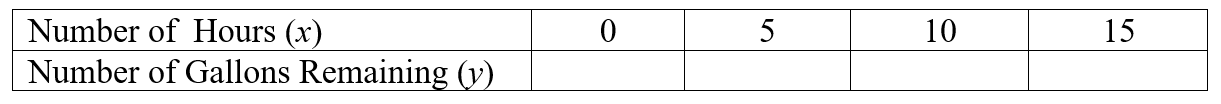# Graphs of Linear Situations

Paul is draining his swimming pool to replace the liner.  Paul’s pool contains $$15,000$$ gallons of water.  He is draining it with a pump that removes $$800$$ gallons per hour.  We can use $$x$$ to represent the number of hours during which the water is pumped and $$y$$ to represent the number of gallons of water remaining in the pool.

1.  What is the slope or rate of change for this linear situation?

Slope:

2.  What is the $$y$$-intercept (or starting point) for this linear situation?

$$y$$-intercept: ( _ , _ )

3. Write an algebraic equation (or function) for this situation.

$$y=$$

## Table

2. Complete the following table to show the number of gallons of water remaining in the pool.## Graph

3. Graph the ordered pairs from the table above on the grid below, and draw a line through the points.## Interpreting the Situation

4. How many hours will it take for the pool to be empty?

5.  What would negative $$y$$-values in the table represent?

Check solutions here.